# The temperature T in a metal ball is inversely proportional to the distance from the center of the ball, which we take to be the origin. The temperature at the point (1, 2, 2) is 110° (a) Find the ra...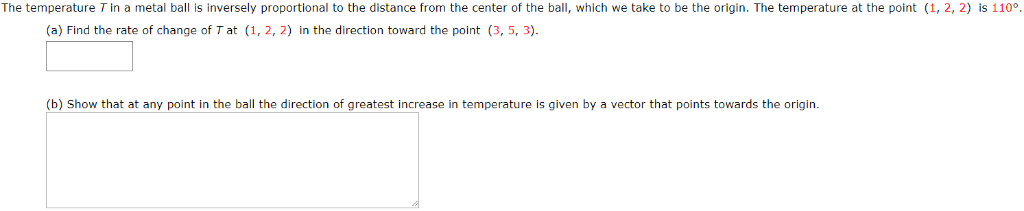The temperature T in a metal ball is inversely proportional to the distance from the center of the ball, which we take to be the origin. The temperature at the point (1, 2, 2) is 110° (a) Find the rate of change of T at (1, 2, 2) in the direction toward the point (3, 5, 3) (b) Show that at any point in the ball the direction of greatest increase in temperature is given by a vector that points towards the origin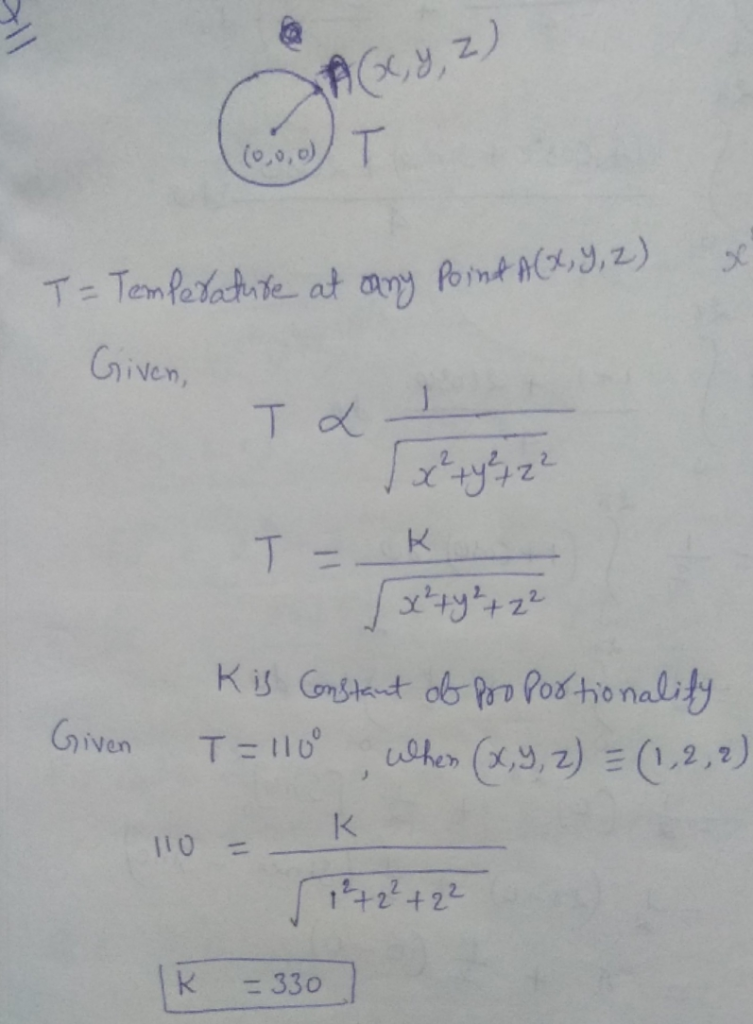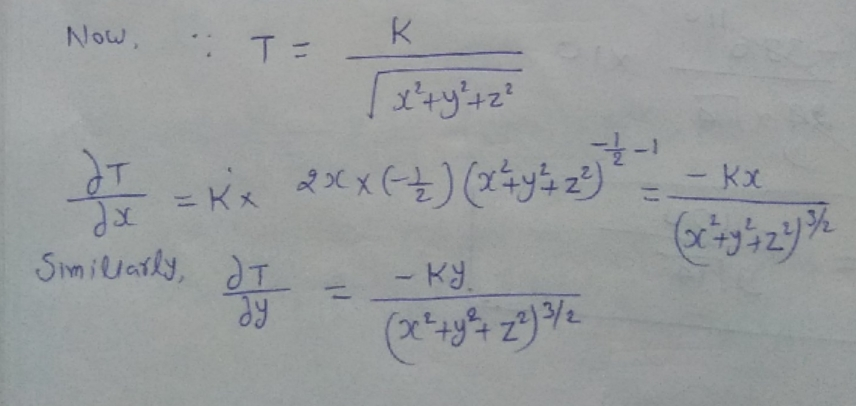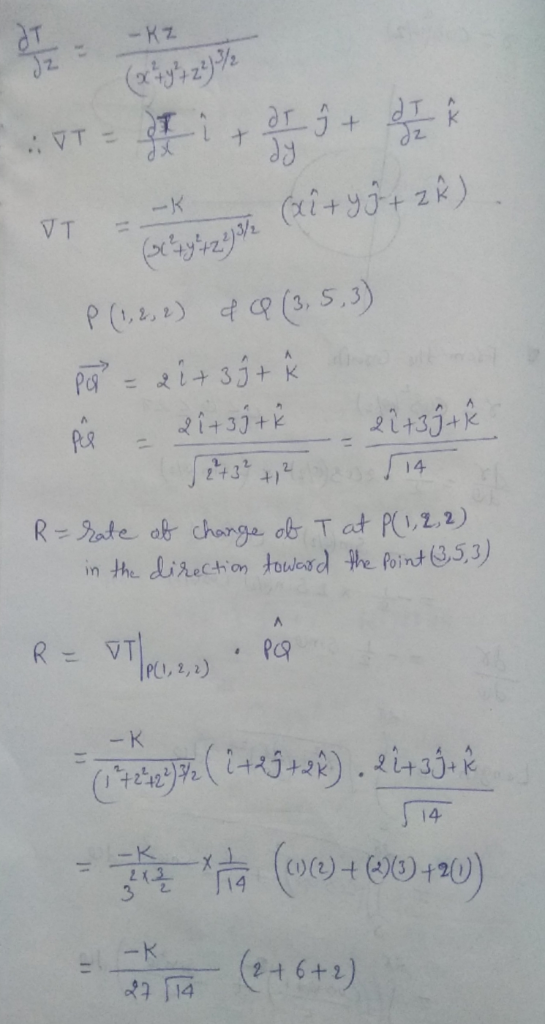##### Add Answer of: The temperature T in a metal ball is inversely proportional to the distance from the center of the ball, which we take to be the origin. The temperature at the point (1, 2, 2) is 110° (a) Find the ra...
Similar Homework Help Questions
• ### 10. The temperature T in a metal ball is inversely proportional to the distance from the center o...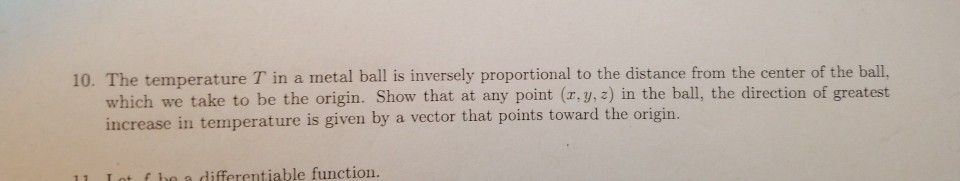10. The temperature T in a metal ball is inversely proportional to the distance from the center of the ball. which we take to be the origin. Show that at any point (r, y, z) in the ball, the direction of greatest increase in temperature is given by a vector that points toward the origin. 11 ot fhe a differentiable function. 10. The temperature T in a metal ball is inversely proportional to the distance from the center of the...

• ### Suppose f is a differentiable function of x and y, and g(r, s) f(8r - s, s2 - 5). Use the table of values below to calc...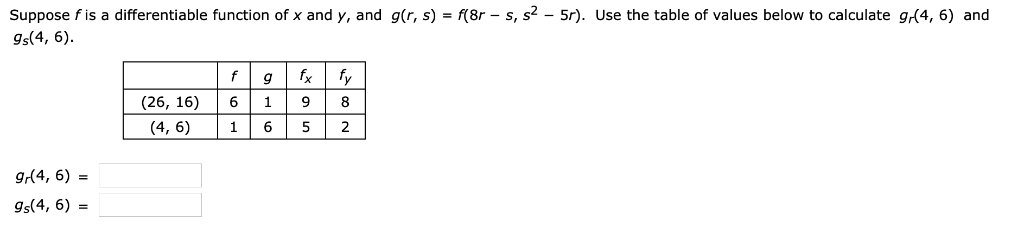Suppose f is a differentiable function of x and y, and g(r, s) f(8r - s, s2 - 5). Use the table of values below to calculate g(4, 6) and s(4, 6) fx (26, 16) 6198 (4, 6)1652 (4, 6) g(4, 6)- The temperature Tin a metal ball is inversely proportional to the distance from the center of the ball, which we take to be the origin. The temperature at the point (2, 1, 2) is 140°K Find DuT, the...

• ### center of mass of lamina (inversely proportional to distance)...

This is a spin off problem 13 in 15.5...problem 13:The boundary of a lamina consists of the semicircles y=√1-x2 and y = √4-x2 together withthe portions of the x-axis that join them.Find the center ofmass of the lamina if the density at any point is proportional toits distance from the origin.Problem 14:Find the center of masas of the lamina in problem 13 if thedeisity at any point is inversely proportional to its distance fromthe origin.I really don't know where to...

• ### The temperature u in a star of conductivity 5 is inversely proportional to the distance from the...

The temperature u in a star of conductivity 5 is inversely proportional to the distance from the center: u = [1/(v{x2 + y2 + z2})].If the star is a sphere of radius 5,find the rate of heat flow outward across the surface of the star.

• ### 5. The temperature of a metal plate at (x,y) is e rate of 8ft/min (.e. -2). degrees. A bug is wal...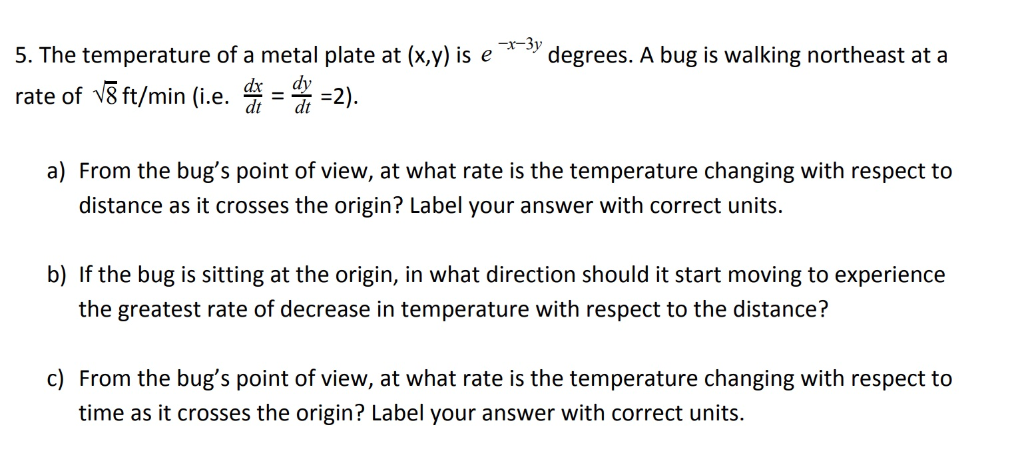5. The temperature of a metal plate at (x,y) is e rate of 8ft/min (.e. -2). degrees. A bug is walking northeast at a dx dy dt dt a) From the bug's point of view, at what rate is the temperature changing with respect to distance as it crosses the origin? Label your answer with correct units. b) If the bug is sitting at the origin, in what direction should it start moving to experience the greatest rate of decrease...

• ### The intensity of a light source at a distance is directly proportional to the strength of the source and inversely proportional to the square of the distance from the source. Two light sources, one f...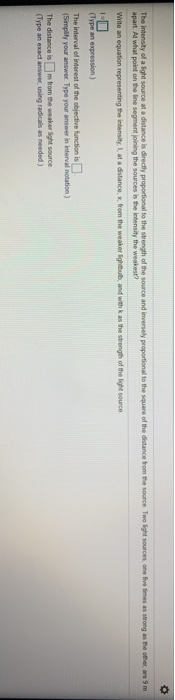The intensity of a light source at a distance is directly proportional to the strength of the source and inversely proportional to the square of the distance from the source. Two light sources, one five times as strong as the other, are 9 m apart. At what point on the line segment joining the sources is the intensity the weakest? Write an equation representing the intensity, I, at a distance, x, from the weaker lightbulb, and with k as the...

• ### (a) The temperature T(x, y) at a point (x, y) on a plate is given by T(x, y) = 16 − x 2 − 2y 2 . ...

(a) The temperature T(x, y) at a point (x, y) on a plate is given by T(x, y) = 16 − x 2 − 2y 2 . i. What is the direction of greatest increase in temperature at the point P = (1, 3)? [3 marks] ii. What are the directions of zero change in temperature at the point P? [4 marks] iii. Find the path of greatest increase in temperature from the point P to the point of maximum...

• ### (1 point) Find the center of mass (r, of the lamina which occupies the region if the density at a...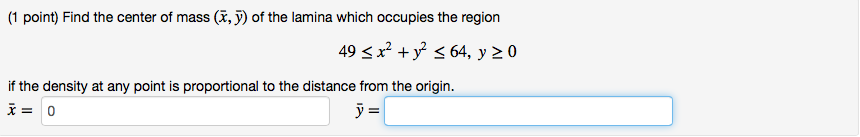how is this done? urgent. (1 point) Find the center of mass (r, of the lamina which occupies the region if the density at any point is proportional to the distance from the origin x= 0 (1 point) Find the center of mass (r, of the lamina which occupies the region if the density at any point is proportional to the distance from the origin x= 0

• ### The brightness of a star is inversely proportional to its distance earth

The brightness of a star is inversely proportional to its distance earthconjecture: the inverse is infinitely large and has an infinite number of stars of equel brightness which are uniformly distributed throughout. Show that this is false( thinkthose stars at a distance between 2^n and 2^n+1 and their contribution to the brightness we see on the earth. Use the fact that it gets dark at night?

• ### The intensity of illumination at a given point is directly proportional to the intensity of the light source and inversely proportional to the square of the distance from the light source

The intensity of illumination at a given point is directly proportional to the intensity of the light source and inversely proportional to the square of the distance from the light source. If a desk is properly illuminated by a 74.0 w lamp 8.00 ft from the desk, what size lamp will be needed to provide the same lighting at a distance of 12.0 ft?

Need Online Homework Help?